# 压电悬臂梁银电极分段发电实验研究Experimental Study on Sectional Power Generation of a Piezoelectric Cantilever Beam with Silver Electrode

• 全文下载: PDF(589KB)    PP.67-73   DOI: 10.12677/AEPE.2018.61007
• 下载量: 521  浏览量: 1,446   国家自然科学基金支持

In order to improve the power generation capacity of a piezoelectric cantilever beam, a method of segmented power generation by the silver electrode of a piezoelectric cantilever beam is proposed in this paper. First, the sectional mode of the piezoelectric vibrator is determined, and the sectional mode is theoretically analyzed, and the expression of the output voltage of each section is obtained. Then the power generation capacity of three different sections of the piezoelectric cantilever beam is tested. The optimum distribution ratio is 1:2:3, and the maximum power generation capacity is 15 V. It provides a theoretical basis and technical reference for the optimal design of a piezoelectric cantilever beam.

1. 引言

2. 压电悬臂梁分段方法

3. 理论分析

${S}_{1}\left(x,t\right)=-\frac{{h}_{c}}{\rho \left(x,t\right)}$ (1)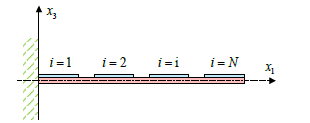Figure 1. Partition method of partition method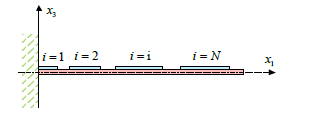Figure 2. Proportion method of electrode section

${D}_{3}\left(x,t\right)=-{d}_{31}{Y}_{p}{h}_{c}\frac{{\partial }^{2}{w}_{rel}\left(x,t\right)}{\partial {x}^{2}}$ (2)

$q\left(t\right)={\int }_{A}D\cdot n\text{d}A=-{\int }_{x={x}_{i}}^{x={x}_{j}}{d}_{31}{Y}_{p}{h}_{c}b\frac{{\partial }^{2}{w}_{rel}\left(x,t\right)}{\partial {x}^{2}}\text{d}x$ (3)

$v\left(t\right)\text{=}-\frac{{d}_{31}{Y}_{p}{h}_{c}b}{{C}_{p}}{\int }_{x={x}_{i}}^{x={x}_{j}}\frac{{\partial }^{2}{w}_{rel}\left(x,t\right)}{\partial {x}^{2}}\text{d}x$ (4)

4. 实验测试

4.1. 测试装置

4.2. 测试分析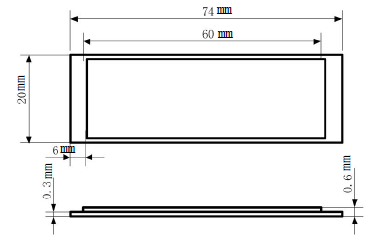Figure 3. Schematic diagram of the structure of a piezoelectric cantilever beam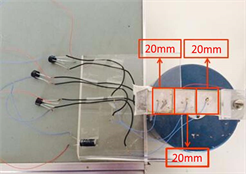Figure 4. Uniform silver electrode power generation device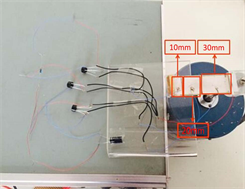Figure 5. Power generation device with a 1:2:3 silver electrode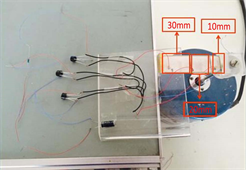Figure 6. Power generation device with a 3:2:1 silver electrodeTable 2. Comparison of generation capacity of silver electrode in subsection

5. 结论

  雷淑梅, 匡同春, 白晓军, 等. 压电陶瓷材料的研究现状与发展趋势[J]. 佛山陶瓷, 2005, 15(3): 36-39.  秦立峰, 陈劭, 宋天佳, 等. 汽车爆胎报警及控制系统的研究现状及发展趋势[J]. 世界科技研究与发展, 2010, 32(4): 501-504.  张永良, 林政. 海洋波浪压电发电技术的进展[C]//中国可再生能源学会海洋能专业委员会第三届学术讨论会论文集. 杭州: 中国可再生能源原学会海洋能专业委员会, 2010.  Szarka, G.D., Stark, B.H. and Burrow, S.G. (2012) Review of Power Conditioning for Kinetic Energy Harvesting Systems. IEEE Transactions on Power Electronics, 27, 803-815. https://doi.org/10.1109/TPEL.2011.2161675  Roundy, S., Wright, P.K. and Rabaey, J. (2003) A Study of Low Level Vibrations as a Power Source for Wireless Sensor Nodes. Computer Commu-nications, 26, 1131-1144. https://doi.org/10.1016/S0140-3664(02)00248-7  Yamagishi, S., Fujimoto, S., Ichiki, M., et al. (2013) Characterization of the Piezoelectric Power Generation of PZT Ceramics under Mechanical Force. 2013 Symposium on Design, Test, Integration and Packaging of MEMS/MOEMS, Barcelona, 1-6.  程光明, 庞建志, 唐可洪, 等. 压电陶瓷发电能力测试系统的研制[J]. 吉林大学学报: 工学版, 2007, 37(2): 367-371.  谢涛, 袁江波, 单小彪, 等. 多悬臂梁压电振子频率分析及发电实验研[J]. 西安交通大学学报, 2010, 44(2): 98-101.  何仁, 胡青训, 薛翔. 汽车轮胎气压监测系统发展综述[J]. 中国安全科学学报, 2006, 15(10): 105-109.  张传忠. 压电材料的发展及应用[J]. 压电与声光, 1993(3): 64-70.  Zhang, L. (2010) Analytical Modeling and Design Optimization of Piezoelectric Bimorph Energy Harvester. The University of Alabama.  Sodano, H.A. and Inman, D.J. (2005) Generation and Storage of Electricity from Power Harvesting Devices. Journal of Intelligent Material Systems & Structures, 16, 67-75. https://doi.org/10.1177/1045389X05047210